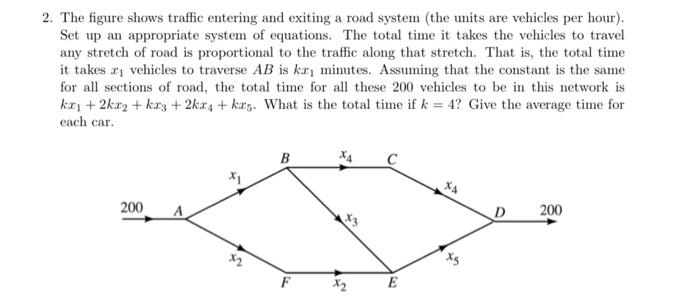# Question 2. The figure shows traffic entering and exiting a road system (the units are vehicles per hour). Set up an appropriate system of equations. The total time it takes the vehicles to travel any stretch of road is proportional to the traffic along that stretch. That is, the total time it takes 11 vehicles to traverse AB is ko minutes. Assuming that the constant is the same for all sections of road, the total time for all these 200 vehicles to be in this network is kr +2kx2 + kr3 +224 + krs. What is the total time if k = 4? Give the average time for each car. B с 200 D 200 X2 F X2 E